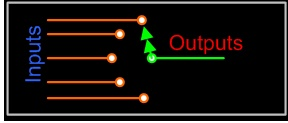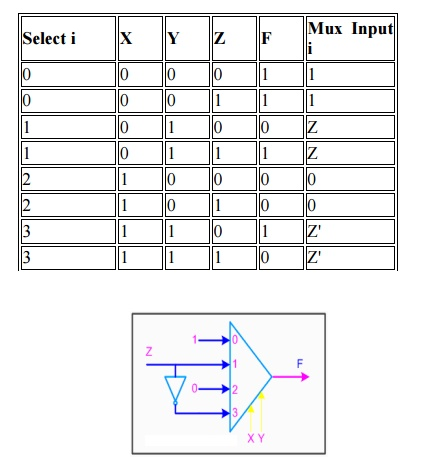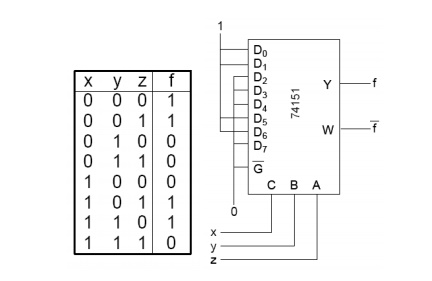Home | | Digital Electronics | Multiplexer

# Multiplexer

A multiplexer (MUX) is a digital switch which connects data from one of n sources to the output.

MULTIPLEXER:

A multiplexer (MUX) is a digital switch which connects data from one of n sources to the output. A number of select inputs determine which data source is connected to the output. The block diagram of MUX with n data sources of b bits wide and s bits wide select line is shown in below figure.MUX acts like a digitally controlled multi-position switch where the binary code applied to the select inputs controls the input source that will be switched on to the output as shown in the figure below. At any given point of time only one input gets selected and is connected to output, based on the select input signal.

The operation of a multiplexer can be better explained using a mechanical switch as shown in the figure below. This rotary switch can touch any of the inputs, which is connected to the output. As you can see at any given point of time only one input gets transferred to output.## 2x1 MUX

A 2 to 1 line multiplexer is shown in figure below, each 2 input lines A to B is applied to one input of an AND gate. Selection lines S are decoded to select a particular AND gate. The truth table for the 2:1 mux is given in the table below.## Design of a 2:1 Mux

To derive the gate level implementation of 2:1 mux we need to have truth table as shown in figure. And once we have the truth table, we can draw the K-map as shown in figure for all the cases when Y is equal to '1'.Combining the two 1' as shown in figure, we can drive the output y as shown below

Y = A.S’ + B.S

### Truth Table## LARGER MULTIPLEXERS

Larger multiplexers can be constructed from smaller ones. An 8-to-1 multiplexer can be constructed from smaller multiplexers as shown below.

### 8-to-1 multiplexer from Smaller MUX### 16-to-1 multiplexer from 4:1 muxIt is 2-to-1 MUX with 4 bits for each input

There is 1 output of 4 bits

There is 1 select signal

When 1 input is selected, the whole group of 4 bits goes to the output### 3-variable Function Using 8-to-1 mux

Implement the function F(X,Y,Z) = S(1,3,5,6) using an 8-to-1 mux. Connect the input variables X, Y, Z to mux select lines. Mux data input lines 1, 3, 5, 6 that correspond to the function minterms are connected to 1. The remaining mux data input lines 0, 2, 4, 7 are connected to 0.### 3-variable Function Using 4-to-1 mux

Implement the function F(X,Y,Z) = S(0,1,3,6) using a single 4-to-1 mux and an inverter. We choose the two most significant inputs X, Y as mux select lines.

### Truth TableWe determine multiplexer input line i values by comparing the remaining input variable Z and the function F for the corresponding selection lines value i

·              when XY=00 the function F is 1 (for both Z=0, Z=1) thus mux input0 = 1

·              when XY=01 the function F is Z thus mux input1 = Z

·              when XY=10 the function F is 0 (for both Z=0, Z=1) thus mux input2 = 0

·              when XY=11 the function F is Z' thus mux input3 = Z'Study Material, Lecturing Notes, Assignment, Reference, Wiki description explanation, brief detail
Digital Electronics : Combinational Circuits : Multiplexer |